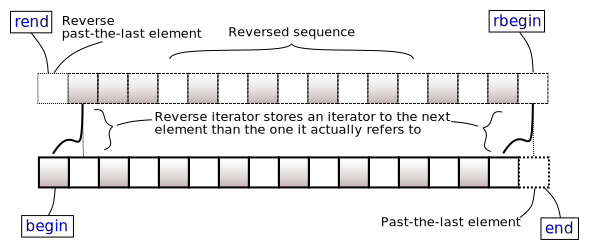/C++

# std::multiset<Key,Compare,Allocator>::rbegin, std::multiset<Key,Compare,Allocator>::crbegin

 (1) `reverse_iterator rbegin();` (until C++11) `reverse_iterator rbegin() noexcept;` (since C++11) (2) `const_reverse_iterator rbegin() const;` (until C++11) `const_reverse_iterator rbegin() const noexcept;` (since C++11) `const_reverse_iterator crbegin() const noexcept;` (3) (since C++11)

Returns a reverse iterator to the first element of the reversed `multiset`. It corresponds to the last element of the non-reversed `multiset`. If the `multiset` is empty, the returned iterator is equal to `rend()`.(none).

### Return value

Reverse iterator to the first element.

### Complexity

Constant.

Because both `iterator` and `const_iterator` are constant iterators (and may in fact be the same type), it is not possible to mutate the elements of the container through an iterator returned by any of these member functions.

### Example

```#include <iostream>
#include <set>

int main()
{
std::multiset<unsigned> rep{1, 2, 3, 4, 1, 2, 3, 4};

for (auto it = rep.crbegin(); it != rep.crend(); ++it)
{
for (auto n = *it; n > 0; --n)
std::cout << "⏼" << ' ';
std::cout << '\n';
}
}```

Output:

```⏼ ⏼ ⏼ ⏼
⏼ ⏼ ⏼ ⏼
⏼ ⏼ ⏼
⏼ ⏼ ⏼
⏼ ⏼
⏼ ⏼
⏼
⏼```